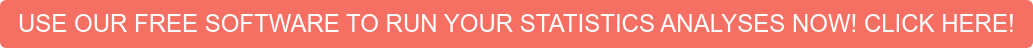# Confirmatory Factor Analysis

Confirmatory factor analysis (CFA) is a multivariate statistical procedure that is used to test how well the measured variables represent the number of constructs.  Confirmatory factor analysis (CFA) and exploratory factor analysis (EFA) are similar techniques, but in exploratory factor analysis (EFA), data is simply explored and provides information about the numbers of factors required to represent the data. In exploratory factor analysis, all measured variables are related to every latent variable.  But in confirmatory factor analysis (CFA), researchers can specify the number of factors required in the data and which measured variable is related to which latent variable.  Confirmatory factor analysis (CFA) is a tool that is used to confirm or reject the measurement theory.General Purpose – Procedure

1. Defining individual construct: First, we have to define the individual constructs. The first step involves the procedure that defines constructs theoretically. This involves a pretest to evaluate the construct items, and a confirmatory test of the measurement model that is conducted using confirmatory factor analysis (CFA), etc.
2. Developing the overall measurement model theory: In confirmatory factor analysis (CFA), we should consider the concept of unidimensionality between construct error variance and within construct error variance.  At least four constructs and three items per constructs should be present in the research.
3. Designing a study to produce the empirical results: The measurement model must be specified.  Most commonly, the value of one loading estimate should be one per construct.  Two methods are available for identification; the first is rank condition, and the second is order condition.
4. Assessing the measurement model validity: Assessing the measurement model validity occurs when the theoretical measurement model is compared with the reality model to see how well the data fits. To check the measurement model validity, the number of the indicator helps us.  For example, the factor loading latent variable should be greater than 0.7.  Chi-square test and other goodness of fit statistics like RMR, GFI, NFI, RMSEA, SIC, BIC, etc., are some key indicators that help in measuring the model validity.

Questions a CFA answers

From my 20 question instrument, are the five factors clearly identifiable constructs as measured by the 4 questions that they are comprised of?

Do my four survey questions accurately measure one factor?

Assumptions

The assumptions of a CFA include multivariate normality, a sufficient sample size (n >200), the correct a priori model specification, and data must come from a random sample.Key Terms:

• Theory: A systematic set of causal relationships that provide the comprehensive explanation of a phenomenon.
• Model: A specified set of dependent relationships that can be used to test the theory.
• Path analysis: Used to test structural equations.
• Path diagram: Shows the graphical representation of cause and effect relationships of the theory.
• Endogenous variable: The resulting variables that are a causal relationship.
• Exogenous variable: The predictor variables.
• Confirmatory analysis: Used to test the pre-specified relationship.
• Cronbach’s alpha: Used to measure the reliability of two or more construct indicators.
• Identification: Used to test whether or not there are a sufficient number of equations to solve the unknown coefficient.  Identifications are of three types: (1) under-identified, (2) exact identified, and (3) over-identified.
• Goodness of fit: The degree to which the observed input matrix is predicted by the estimated model.
• Latent variables: Variables that are inferred, not directly observed, from other variables that are observed.

Confirmatory factor analysis (CFA) and statistical software:

Usually, statistical software like AMOS, LISREL, EQS and SAS are used for confirmatory factor analysis.  In AMOS, visual paths are manually drawn on the graphic window and analysis is performed. In LISREL, confirmatory factor analysis can be performed graphically as well as from the menu.  In SAS, confirmatory factor analysis can be performed by using the programming languages.

Related Pages: